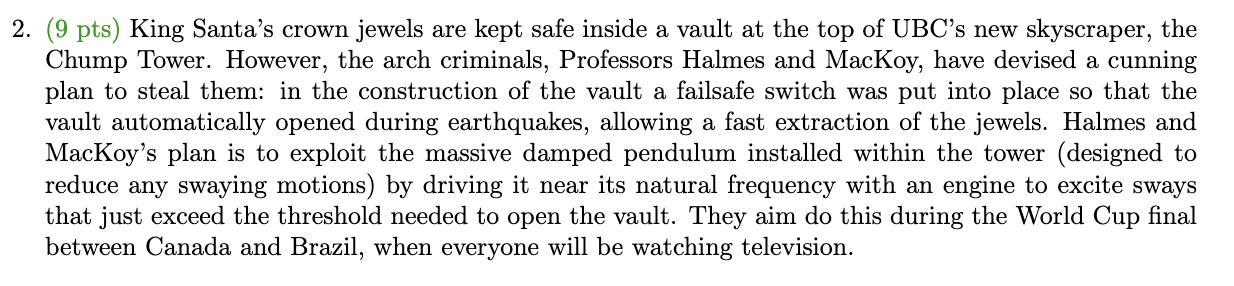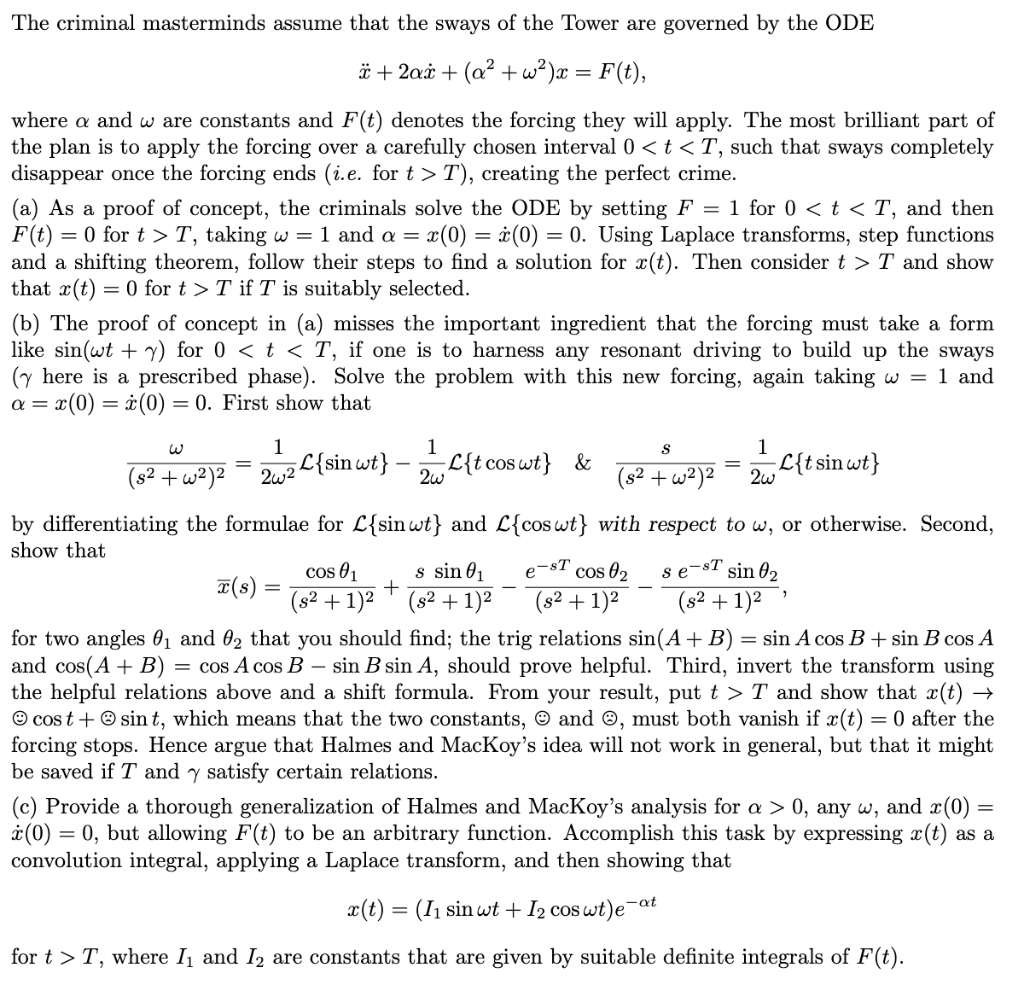# Question (9 pts) King Santa's crown jewels are kept safe inside a vault at the top of UBC's new skyscraper, the Chump Tower. However, the arch criminals, Professors Halmes and MacKoy, have devised a cunning plan to steal them: in the construction of the vault a failsafe switch was put into place so that the vault automatically opened during earthquakes, allowing a fast extraction of the jewels. Halmes and MacKoy's plan is to exploit the massive damped pendulum installed within the tower (designed to reduce any swaying motions) by driving it near its natural frequency with an engine to excite sways that just exceed the threshold needed to open the vault. They aim do this during the World Cup final between Canada and Brazil, when everyone will be watching television. $\ddot{x}+2 \alpha \dot{x}+\left(\alpha^{2}+\omega^{2}\right) x=F(t),$ where $$\alpha$$ and $$\omega$$ are constants and $$F(t)$$ denotes the forcing they will apply. The most brilliant part of the plan is to apply the forcing over a carefully chosen interval $$0<t<T$$, such that sways completely disappear once the forcing ends (i.e. for $$t>T)$$, creating the perfect crime. (a) As a proof of concept, the criminals solve the ODE by setting $$F=1$$ for $$0<t<T$$, and then $$F(t)=0$$ for $$t>T$$, taking $$\omega=1$$ and $$\alpha=x(0)=\dot{x}(0)=0$$. Using Laplace transforms, step functions and a shifting theorem, follow their steps to find a solution for $$x(t)$$. Then consider $$t>T$$ and show that $$x(t)=0$$ for $$t>T$$ if $$T$$ is suitably selected. (b) The proof of concept in (a) misses the important ingredient that the forcing must take a form like $$\sin (\omega t+\gamma)$$ for $$0<t<T$$, if one is to harness any resonant driving to build up the sways ( $$\gamma$$ here is a prescribed phase). Solve the problem with this new forcing, again taking $$\omega=1$$ and $$\alpha=x(0)=\dot{x}(0)=0$$. First show that $\frac{\omega}{\left(s^{2}+\omega^{2}\right)^{2}}=\frac{1}{2 \omega^{2}} \mathcal{L}\{\sin \omega t\}-\frac{1}{2 \omega} \mathcal{L}\{t \cos \omega t\} \quad \& \quad \frac{s}{\left(s^{2}+\omega^{2}\right)^{2}}=\frac{1}{2 \omega} \mathcal{L}\{t \sin \omega t\}$ by differentiating the formulae for $$\mathcal{L}\{\sin \omega t\}$$ and $$\mathcal{L}\{\cos \omega t\}$$ with respect to $$\omega$$, or otherwise. Second, show that $\bar{x}(s)=\frac{\cos \theta_{1}}{\left(s^{2}+1\right)^{2}}+\frac{s \sin \theta_{1}}{\left(s^{2}+1\right)^{2}}-\frac{e^{-s T} \cos \theta_{2}}{\left(s^{2}+1\right)^{2}}-\frac{s e^{-s T} \sin \theta_{2}}{\left(s^{2}+1\right)^{2}}$ for two angles $$\theta_{1}$$ and $$\theta_{2}$$ that you should find; the trig relations $$\sin (A+B)=\sin A \cos B+\sin B \cos A$$ and $$\cos (A+B)=\cos A \cos B-\sin B \sin A$$, should prove helpful. Third, invert the transform using the helpful relations above and a shift formula. From your result, put $$t>T$$ and show that $$x(t) \rightarrow$$ (-) $$\cos t+\otimes \sin t$$, which means that the two constants, $$\odot$$ and $$\odot$$, must both vanish if $$x(t)=0$$ after the forcing stops. Hence argue that Halmes and MacKoy's idea will not work in general, but that it might be saved if $$T$$ and $$\gamma$$ satisfy certain relations. (c) Provide a thorough generalization of Halmes and MacKoy's analysis for $$\alpha>0$$, any $$\omega$$, and $$x(0)=$$ $$\dot{x}(0)=0$$, but allowing $$F(t)$$ to be an arbitrary function. Accomplish this task by expressing $$x(t)$$ as a convolution integral, applying a Laplace transform, and then showing that $x(t)=\left(I_{1} \sin \omega t+I_{2} \cos \omega t\right) e^{-\alpha t}$ for $$t>T$$, where $$I_{1}$$ and $$I_{2}$$ are constants that are given by suitable definite integrals of $$F(t)$$.Transcribed Image Text: (9 pts) King Santa's crown jewels are kept safe inside a vault at the top of UBC's new skyscraper, the Chump Tower. However, the arch criminals, Professors Halmes and MacKoy, have devised a cunning plan to steal them: in the construction of the vault a failsafe switch was put into place so that the vault automatically opened during earthquakes, allowing a fast extraction of the jewels. Halmes and MacKoy's plan is to exploit the massive damped pendulum installed within the tower (designed to reduce any swaying motions) by driving it near its natural frequency with an engine to excite sways that just exceed the threshold needed to open the vault. They aim do this during the World Cup final between Canada and Brazil, when everyone will be watching television. $\ddot{x}+2 \alpha \dot{x}+\left(\alpha^{2}+\omega^{2}\right) x=F(t),$ where $$\alpha$$ and $$\omega$$ are constants and $$F(t)$$ denotes the forcing they will apply. The most brilliant part of the plan is to apply the forcing over a carefully chosen interval $$0T)$$, creating the perfect crime. (a) As a proof of concept, the criminals solve the ODE by setting $$F=1$$ for $$0T$$, taking $$\omega=1$$ and $$\alpha=x(0)=\dot{x}(0)=0$$. Using Laplace transforms, step functions and a shifting theorem, follow their steps to find a solution for $$x(t)$$. Then consider $$t>T$$ and show that $$x(t)=0$$ for $$t>T$$ if $$T$$ is suitably selected. (b) The proof of concept in (a) misses the important ingredient that the forcing must take a form like $$\sin (\omega t+\gamma)$$ for $$0T$$ and show that $$x(t) \rightarrow$$ (-) $$\cos t+\otimes \sin t$$, which means that the two constants, $$\odot$$ and $$\odot$$, must both vanish if $$x(t)=0$$ after the forcing stops. Hence argue that Halmes and MacKoy's idea will not work in general, but that it might be saved if $$T$$ and $$\gamma$$ satisfy certain relations. (c) Provide a thorough generalization of Halmes and MacKoy's analysis for $$\alpha>0$$, any $$\omega$$, and $$x(0)=$$ $$\dot{x}(0)=0$$, but allowing $$F(t)$$ to be an arbitrary function. Accomplish this task by expressing $$x(t)$$ as a convolution integral, applying a Laplace transform, and then showing that $x(t)=\left(I_{1} \sin \omega t+I_{2} \cos \omega t\right) e^{-\alpha t}$ for $$t>T$$, where $$I_{1}$$ and $$I_{2}$$ are constants that are given by suitable definite integrals of $$F(t)$$.
Transcribed Image Text: (9 pts) King Santa's crown jewels are kept safe inside a vault at the top of UBC's new skyscraper, the Chump Tower. However, the arch criminals, Professors Halmes and MacKoy, have devised a cunning plan to steal them: in the construction of the vault a failsafe switch was put into place so that the vault automatically opened during earthquakes, allowing a fast extraction of the jewels. Halmes and MacKoy's plan is to exploit the massive damped pendulum installed within the tower (designed to reduce any swaying motions) by driving it near its natural frequency with an engine to excite sways that just exceed the threshold needed to open the vault. They aim do this during the World Cup final between Canada and Brazil, when everyone will be watching television. $\ddot{x}+2 \alpha \dot{x}+\left(\alpha^{2}+\omega^{2}\right) x=F(t),$ where $$\alpha$$ and $$\omega$$ are constants and $$F(t)$$ denotes the forcing they will apply. The most brilliant part of the plan is to apply the forcing over a carefully chosen interval $$0T)$$, creating the perfect crime. (a) As a proof of concept, the criminals solve the ODE by setting $$F=1$$ for $$0T$$, taking $$\omega=1$$ and $$\alpha=x(0)=\dot{x}(0)=0$$. Using Laplace transforms, step functions and a shifting theorem, follow their steps to find a solution for $$x(t)$$. Then consider $$t>T$$ and show that $$x(t)=0$$ for $$t>T$$ if $$T$$ is suitably selected. (b) The proof of concept in (a) misses the important ingredient that the forcing must take a form like $$\sin (\omega t+\gamma)$$ for $$0T$$ and show that $$x(t) \rightarrow$$ (-) $$\cos t+\otimes \sin t$$, which means that the two constants, $$\odot$$ and $$\odot$$, must both vanish if $$x(t)=0$$ after the forcing stops. Hence argue that Halmes and MacKoy's idea will not work in general, but that it might be saved if $$T$$ and $$\gamma$$ satisfy certain relations. (c) Provide a thorough generalization of Halmes and MacKoy's analysis for $$\alpha>0$$, any $$\omega$$, and $$x(0)=$$ $$\dot{x}(0)=0$$, but allowing $$F(t)$$ to be an arbitrary function. Accomplish this task by expressing $$x(t)$$ as a convolution integral, applying a Laplace transform, and then showing that $x(t)=\left(I_{1} \sin \omega t+I_{2} \cos \omega t\right) e^{-\alpha t}$ for $$t>T$$, where $$I_{1}$$ and $$I_{2}$$ are constants that are given by suitable definite integrals of $$F(t)$$.